# Ac fundamentals

27. May 2014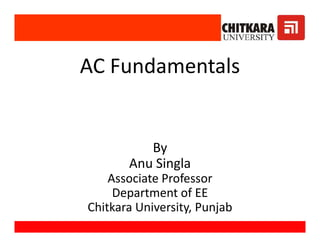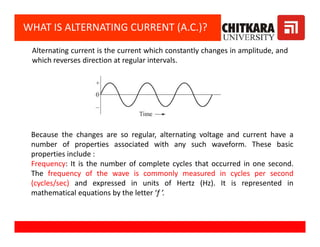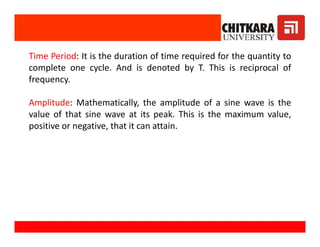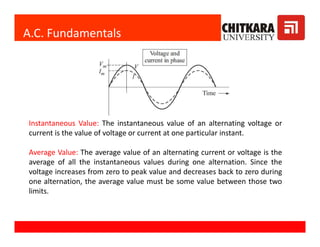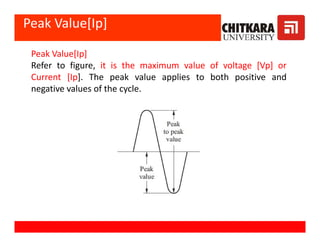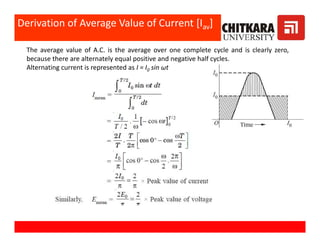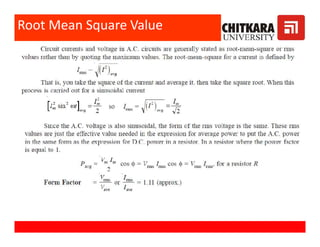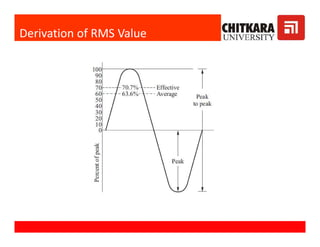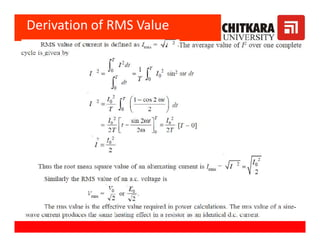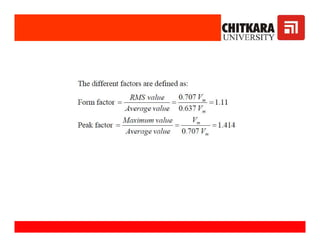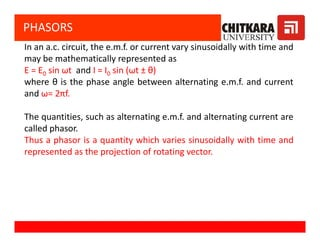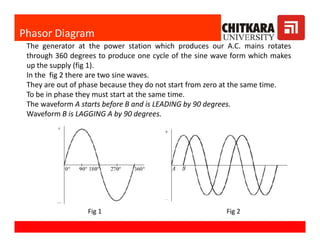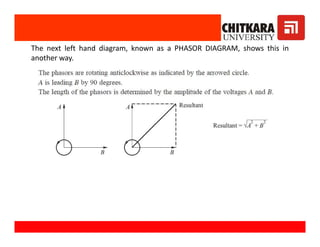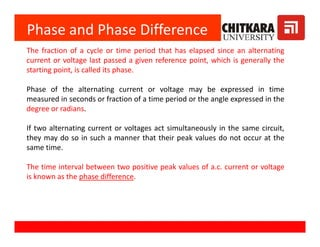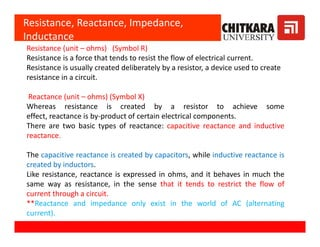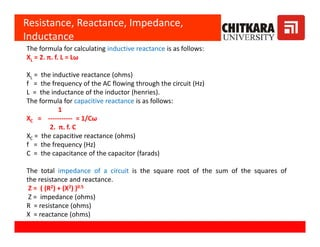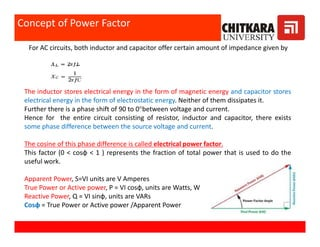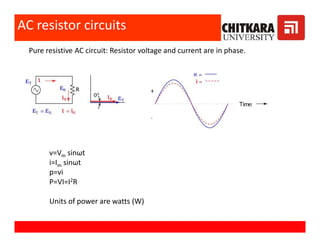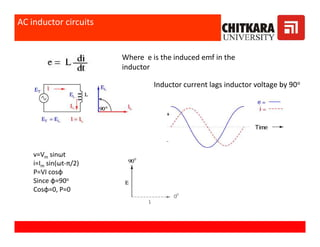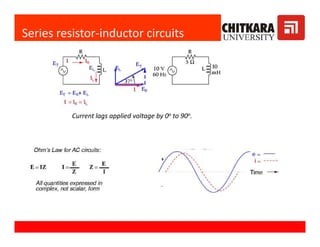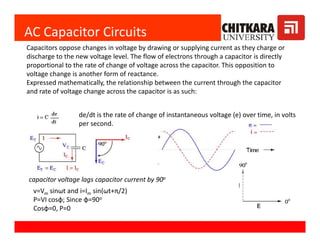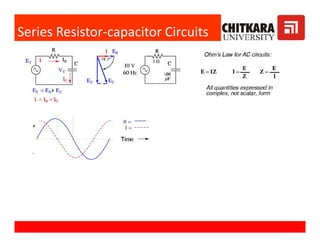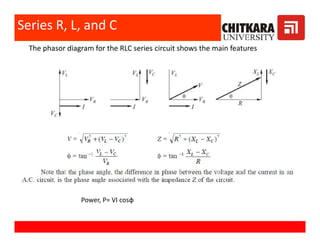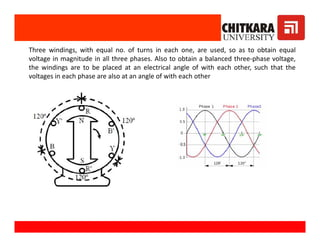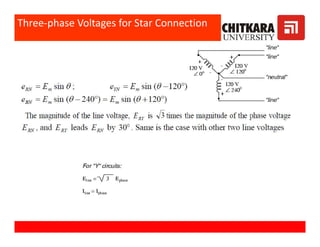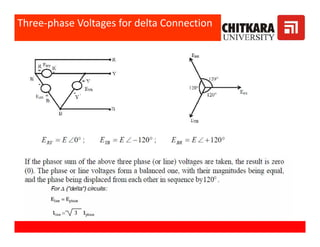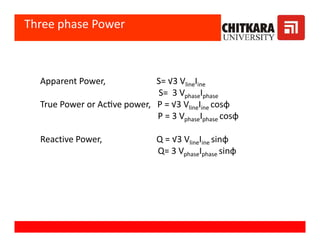1 von 28

### Ac fundamentals

• 1. AC Fundamentals By Anu Singla Associate Professor Department of EE Chitkara University, Punjab AC Fundamentals By Anu Singla Associate Professor Department of EE Chitkara University, Punjab
• 2. WHAT IS ALTERNATING CURRENT (A.C.)? Alternating current is the current which constantly changes in amplitude, and which reverses direction at regular intervals. Because the changes are so regular, alternating voltage and current have a number of properties associated with any such waveform. These basic properties include : Frequency: It is the number of complete cycles that occurred in one second. The frequency of the wave is commonly measured in cycles per second (cycles/sec) and expressed in units of Hertz (Hz). It is represented in mathematical equations by the letter ‘f ’.
• 3. Time Period: It is the duration of time required for the quantity to complete one cycle. And is denoted by T. This is reciprocal of frequency. Amplitude: Mathematically, the amplitude of a sine wave is the value of that sine wave at its peak. This is the maximum value, positive or negative, that it can attain. Time Period: It is the duration of time required for the quantity to complete one cycle. And is denoted by T. This is reciprocal of frequency. Amplitude: Mathematically, the amplitude of a sine wave is the value of that sine wave at its peak. This is the maximum value, positive or negative, that it can attain.
• 4. A.C. Fundamentals Instantaneous Value: The instantaneous value of an alternating voltage or current is the value of voltage or current at one particular instant. Average Value: The average value of an alternating current or voltage is the average of all the instantaneous values during one alternation. Since the voltage increases from zero to peak value and decreases back to zero during one alternation, the average value must be some value between those two limits. Instantaneous Value: The instantaneous value of an alternating voltage or current is the value of voltage or current at one particular instant. Average Value: The average value of an alternating current or voltage is the average of all the instantaneous values during one alternation. Since the voltage increases from zero to peak value and decreases back to zero during one alternation, the average value must be some value between those two limits.
• 5. Peak Value[Ip] Peak Value[Ip] Refer to figure, it is the maximum value of voltage [Vp] or Current [Ip]. The peak value applies to both positive and negative values of the cycle.
• 6. The average value of A.C. is the average over one complete cycle and is clearly zero, because there are alternately equal positive and negative half cycles. Alternating current is represented as I = I0 sin ωt Derivation of Average Value of Current [Iav]
• 11. PHASORS In an a.c. circuit, the e.m.f. or current vary sinusoidally with time and may be mathematically represented as E = E0 sin ωt and I = I0 sin (ωt ± θ) where θ is the phase angle between alternating e.m.f. and current and ω= 2πf. The quantities, such as alternating e.m.f. and alternating current are called phasor. Thus a phasor is a quantity which varies sinusoidally with time and represented as the projection of rotating vector. In an a.c. circuit, the e.m.f. or current vary sinusoidally with time and may be mathematically represented as E = E0 sin ωt and I = I0 sin (ωt ± θ) where θ is the phase angle between alternating e.m.f. and current and ω= 2πf. The quantities, such as alternating e.m.f. and alternating current are called phasor. Thus a phasor is a quantity which varies sinusoidally with time and represented as the projection of rotating vector.
• 12. Phasor Diagram The generator at the power station which produces our A.C. mains rotates through 360 degrees to produce one cycle of the sine wave form which makes up the supply (fig 1). In the fig 2 there are two sine waves. They are out of phase because they do not start from zero at the same time. To be in phase they must start at the same time. The waveform A starts before B and is LEADING by 90 degrees. Waveform B is LAGGING A by 90 degrees. Fig 1 Fig 2
• 13. The next left hand diagram, known as a PHASOR DIAGRAM, shows this in another way.
• 14. Phase and Phase Difference The fraction of a cycle or time period that has elapsed since an alternating current or voltage last passed a given reference point, which is generally the starting point, is called its phase. Phase of the alternating current or voltage may be expressed in time measured in seconds or fraction of a time period or the angle expressed in the degree or radians. If two alternating current or voltages act simultaneously in the same circuit, they may do so in such a manner that their peak values do not occur at the same time. The time interval between two positive peak values of a.c. current or voltage is known as the phase difference. The fraction of a cycle or time period that has elapsed since an alternating current or voltage last passed a given reference point, which is generally the starting point, is called its phase. Phase of the alternating current or voltage may be expressed in time measured in seconds or fraction of a time period or the angle expressed in the degree or radians. If two alternating current or voltages act simultaneously in the same circuit, they may do so in such a manner that their peak values do not occur at the same time. The time interval between two positive peak values of a.c. current or voltage is known as the phase difference.
• 15. Resistance, Reactance, Impedance, Inductance Resistance (unit – ohms) (Symbol R) Resistance is a force that tends to resist the flow of electrical current. Resistance is usually created deliberately by a resistor, a device used to create resistance in a circuit. Reactance (unit – ohms) (Symbol X) Whereas resistance is created by a resistor to achieve some effect, reactance is by-product of certain electrical components. There are two basic types of reactance: capacitive reactance and inductive reactance. The capacitive reactance is created by capacitors, while inductive reactance is created by inductors. Like resistance, reactance is expressed in ohms, and it behaves in much the same way as resistance, in the sense that it tends to restrict the flow of current through a circuit. **Reactance and impedance only exist in the world of AC (alternating current). Resistance (unit – ohms) (Symbol R) Resistance is a force that tends to resist the flow of electrical current. Resistance is usually created deliberately by a resistor, a device used to create resistance in a circuit. Reactance (unit – ohms) (Symbol X) Whereas resistance is created by a resistor to achieve some effect, reactance is by-product of certain electrical components. There are two basic types of reactance: capacitive reactance and inductive reactance. The capacitive reactance is created by capacitors, while inductive reactance is created by inductors. Like resistance, reactance is expressed in ohms, and it behaves in much the same way as resistance, in the sense that it tends to restrict the flow of current through a circuit. **Reactance and impedance only exist in the world of AC (alternating current).
• 16. The formula for calculating inductive reactance is as follows: XL = 2. π. f. L = Lω XL = the inductive reactance (ohms) f = the frequency of the AC flowing through the circuit (Hz) L = the inductance of the inductor (henries). The formula for capacitive reactance is as follows: 1 XC = ----------- = 1/Cω 2. π. f. C XC = the capacitive reactance (ohms) f = the frequency (Hz) C = the capacitance of the capacitor (farads) The total impedance of a circuit is the square root of the sum of the squares of the resistance and reactance. Z = ( (R2) + (X2) )0.5 Z = impedance (ohms) R = resistance (ohms) X = reactance (ohms) Resistance, Reactance, Impedance, Inductance The formula for calculating inductive reactance is as follows: XL = 2. π. f. L = Lω XL = the inductive reactance (ohms) f = the frequency of the AC flowing through the circuit (Hz) L = the inductance of the inductor (henries). The formula for capacitive reactance is as follows: 1 XC = ----------- = 1/Cω 2. π. f. C XC = the capacitive reactance (ohms) f = the frequency (Hz) C = the capacitance of the capacitor (farads) The total impedance of a circuit is the square root of the sum of the squares of the resistance and reactance. Z = ( (R2) + (X2) )0.5 Z = impedance (ohms) R = resistance (ohms) X = reactance (ohms)
• 17. For AC circuits, both inductor and capacitor offer certain amount of impedance given by The inductor stores electrical energy in the form of magnetic energy and capacitor stores electrical energy in the form of electrostatic energy. Neither of them dissipates it. Further there is a phase shift of 90 to 0°between voltage and current. Hence for the entire circuit consisting of resistor, inductor and capacitor, there exists some phase difference between the source voltage and current. The cosine of this phase difference is called electrical power factor. This factor (0 < cosφ < 1 ) represents the fraction of total power that is used to do the useful work. Apparent Power, S=VI units are V Amperes True Power or Active power, P = VI cosφ, units are Watts, W Reactive Power, Q = VI sinφ, units are VARs Cosφ = True Power or Active power /Apparent Power Concept of Power Factor The inductor stores electrical energy in the form of magnetic energy and capacitor stores electrical energy in the form of electrostatic energy. Neither of them dissipates it. Further there is a phase shift of 90 to 0°between voltage and current. Hence for the entire circuit consisting of resistor, inductor and capacitor, there exists some phase difference between the source voltage and current. The cosine of this phase difference is called electrical power factor. This factor (0 < cosφ < 1 ) represents the fraction of total power that is used to do the useful work. Apparent Power, S=VI units are V Amperes True Power or Active power, P = VI cosφ, units are Watts, W Reactive Power, Q = VI sinφ, units are VARs Cosφ = True Power or Active power /Apparent Power
• 18. AC resistor circuits Pure resistive AC circuit: Resistor voltage and current are in phase. v=Vm sinωt i=Im sinωt p=vi P=VI=I2R Units of power are watts (W)
• 19. AC inductor circuits Where e is the induced emf in the inductor Inductor current lags inductor voltage by 90o v=Vm sinωt i=Im sin(ωt-π/2) P=VI cosφ Since φ=90o Cosφ=0, P=0
• 20. Current lags applied voltage by 0o to 90o. Series resistor-inductor circuits
• 21. AC Capacitor Circuits Capacitors oppose changes in voltage by drawing or supplying current as they charge or discharge to the new voltage level. The flow of electrons through a capacitor is directly proportional to the rate of change of voltage across the capacitor. This opposition to voltage change is another form of reactance. Expressed mathematically, the relationship between the current through the capacitor and rate of voltage change across the capacitor is as such: de/dt is the rate of change of instantaneous voltage (e) over time, in volts per second. de/dt is the rate of change of instantaneous voltage (e) over time, in volts per second. capacitor voltage lags capacitor current by 90o v=Vm sinωt and i=Im sin(ωt+π/2) P=VI cosφ; Since φ=90o Cosφ=0, P=0
• 23. The phasor diagram for the RLC series circuit shows the main features Series R, L, and C Power, P= VI cosφ
• 24. Three windings, with equal no. of turns in each one, are used, so as to obtain equal voltage in magnitude in all three phases. Also to obtain a balanced three-phase voltage, the windings are to be placed at an electrical angle of with each other, such that the voltages in each phase are also at an angle of with each other
• 25. Three-phase Voltages for Star Connection
• 26. Three-phase Voltages for delta Connection
• 27. Apparent Power, S= √3 VlineIine S= 3 VphaseIphase True Power or Ac ve power, P = √3 VlineIine cosφ P = 3 VphaseIphase cosφ Reactive Power, Q = √3 VlineIine sinφ Q= 3 VphaseIphase sinφ Three phase Power Apparent Power, S= √3 VlineIine S= 3 VphaseIphase True Power or Ac ve power, P = √3 VlineIine cosφ P = 3 VphaseIphase cosφ Reactive Power, Q = √3 VlineIine sinφ Q= 3 VphaseIphase sinφ